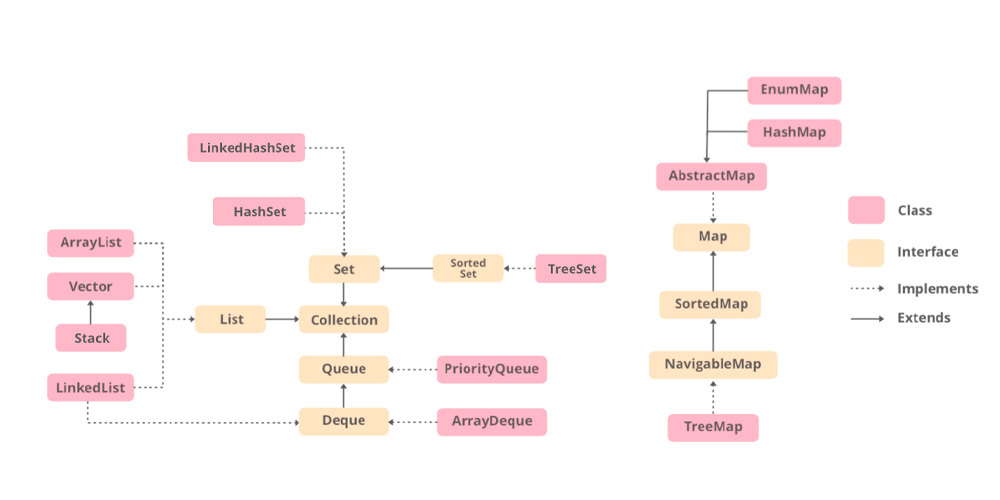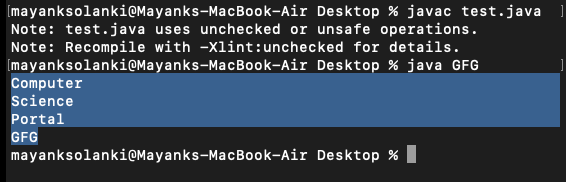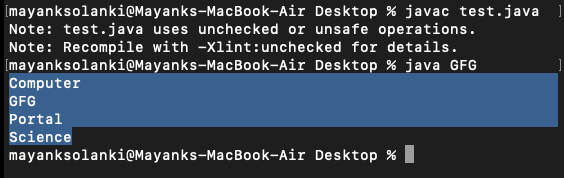Open in App
Not now

# HashSet vs TreeSet in Java

• Difficulty Level : Easy
• Last Updated : 02 Feb, 2023

When it comes to discussing differences between Set the firstmost thing that comes into play is the insertion order and how elements will be processed. HashSet in java is a class implementing the Set interface, backed by a hash table which is actually a HashMap instance. This class permits the null element. The class also offers constant time performance for the basic operations like add, remove, contains, and size assuming the hash function disperses the elements properly among the buckets while TreeSet is an implementation of the SortedSet interface which as the name suggests uses the tree for storage purposes where here the ordering of the elements is maintained by a set using their natural ordering whether or not an explicit comparator is provided.### 1. Speed and internal implementation

For operations like search, insert, and delete HashSet takes constant time for these operations on average. HashSet is faster than TreeSet. HashSet is Implemented using a hash table. TreeSet takes O(Log n) for search, insert and delete which is higher than HashSet. But TreeSet keeps sorted data. Also, it supports operations like higher() (Returns least higher element), floor(), ceiling(), etc. These operations are also O(Log n) in TreeSet and not supported in HashSet. TreeSet is implemented using a self-balancing binary search tree (Red-Black Tree). TreeSet is backed by TreeMap in Java.

### 2. Ordering

Elements in HashSet are not ordered. TreeSet maintains objects in Sorted order defined by either Comparable or Comparator method in Java. TreeSet elements are sorted in ascending order by default. It offers several methods to deal with the ordered set like first(), last(), headSet(), tailSet(), etc.

### 3. Null Object

HashSet allows null object. TreeSet doesn’t allow null Object and throw NullPointerException, Why, because TreeSet uses compareTo() method to compare keys and compareTo() will throw java.lang.NullPointerException.

### 4. Comparison

HashSet uses the equals() method to compare two objects in Set and for detecting duplicates. TreeSet uses compareTo() method for same purpose. If equals() and compareTo() are not consistent, i.e. for two equal objects equals should return true while compareTo() should return zero, then it will break the contract of Set interface and will allow duplicates in Set implementations like TreeSet

Note: If you want a sorted Set then it is better to add elements to HashSet and then convert it into TreeSet rather than creating a TreeSet and adding elements to it.

Geek after going through their differences now you must be wondering when to prefer TreeSet over HashSet?

1. Sorted unique elements are required instead of unique elements. The sorted list given by TreeSet is always in ascending order.
2. TreeSet has a greater locality than HashSet.If two entries are nearby in the order, then TreeSet places them near each other in data structure and hence in memory, while HashSet spreads the entries all over memory regardless of the keys they are associated to.
3. TreeSet uses a Red-Black tree algorithm underneath to sort out the elements. When one needs to perform read/write operations frequently, then TreeSet is a good choice.
4. LinkedHashSet is another data structure that is between these two. It provides time complexities like HashSet and maintains the order of insertion (Note that this is not sorted order, but the order in which elements are inserted).

Implementation: Here we will be discussing them both with 2 examples for both of them. Let us start with HashSet later on dwelling on to TreeSet.

• HashSet examples
• TreeSet examples

Example 1:

## Java

 `// Java Program to Demonstrate Working of HashSet Class` `// Importing required HashSet class from java.util package``import` `java.util.HashSet;` `// Main class``class` `GFG {` `    ``// Main driver method``    ``public` `static` `void` `main(String[] args)``    ``{``        ``// Creating a HashSet object of string type``        ``HashSet hset = ``new` `HashSet();` `        ``// Adding elements to HashSet``        ``// using add() method``        ``hset.add(``"geeks"``);``        ``hset.add(``"for"``);``        ``hset.add(``"practice"``);``        ``hset.add(``"contribute"``);` `        ``// Duplicate removed``        ``hset.add(``"geeks"``);` `        ``// Printing HashSet elements using for each loop` `        ``// Display message only``        ``System.out.println(``"HashSet contains: "``);` `        ``// Iterating over hashSet using for-each loop``        ``for` `(String temp : hset) {` `            ``// Printing all elements inside above hashSet``            ``System.out.println(temp);``        ``}``    ``}``}`

Output:

```HashSet contains:
practice
geeks
for
contribute```

Example 2:

## Java

 `// Java Program to Illustrate Working of HashSet Class``// From another Collection` `// Importing utility classes``import` `java.util.*;` `class` `GFG {` `    ``// Main driver method``    ``public` `static` `void` `main(String[] args)``    ``{` `        ``ArrayList ll = ``new` `ArrayList();` `        ``// Adding elements to ArrayList``        ``ll.add(``"Computer"``);``        ``ll.add(``"Science"``);` `        ``// Creating HashSet object of string type``        ``HashSet hs = ``new` `HashSet(ll);``        ``hs.add(``"Portal"``);``        ``hs.add(``"GFG"``);` `        ``// Iterating via iterators``        ``Iterator iter = hs.iterator();` `        ``// Condition holds true till there is single element``        ``// in the List``        ``while` `(iter.hasNext()) {` `            ``// Printing all elements inside objects``            ``System.out.println(iter.next());``        ``}``    ``}``}`

Output:Now it’s time to implement TreeSet to understand better via implementing it.

Example 1:

## Java

 `// Java program to demonstrate working of``// TreeSet Class` `// Importing required classes``import` `java.util.TreeSet;` `// Class``class` `TreeSetDemo {` `    ``// Main driver method``    ``public` `static` `void` `main(String[] args)``    ``{``        ``// Create an empty TreeSet``        ``TreeSet tset = ``new` `TreeSet();` `        ``// Adding elements to HashSet``        ``// using add() method``        ``tset.add(``"geeks"``);``        ``tset.add(``"for"``);``        ``tset.add(``"practice"``);``        ``tset.add(``"contribute"``);` `        ``// Duplicate elements being removed``        ``tset.add(``"geeks"``);` `        ``// Displaying TreeSet elements``        ``System.out.println(``"TreeSet contains: "``);``      ` `        ``for` `(String temp : tset) {``            ``System.out.println(temp);``        ``}``    ``}``}`

Output:

```TreeSet contains:
contribute
for
geeks
practice```

Example 2:

## Java

 `// Java Program to Illustrate Working of TreeSet``// From another Collection` `// Importing utility classes``import` `java.util.*;` `// Main class``class` `GFG {` `    ``// Main driver method``    ``public` `static` `void` `main(String[] args)``    ``{` `        ``// Creating list of string``        ``ArrayList ll = ``new` `ArrayList();` `        ``// Adding elements to ArrayList``        ``// using add() method``        ``ll.add(``"Computer"``);``        ``ll.add(``"Science"``);` `        ``// Creating TreeSet object of string type``        ``TreeSet ts = ``new` `TreeSet(ll);` `        ``// Adding elements to above TreeSet``        ``ts.add(``"Portal"``);``        ``ts.add(``"GFG"``);` `        ``// Iterating via iterators``        ``Iterator iter = ts.iterator();` `        ``// Condition that holds true till``        ``// there is single element in the List``        ``while` `(iter.hasNext()) {` `            ``// Printing all elements inside objects``            ``System.out.println(iter.next());``        ``}``    ``}``}`

Output:My Personal Notes arrow_drop_up